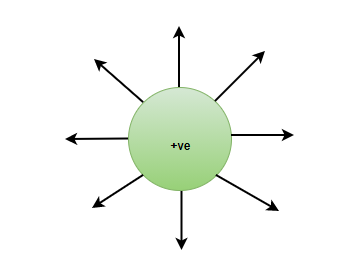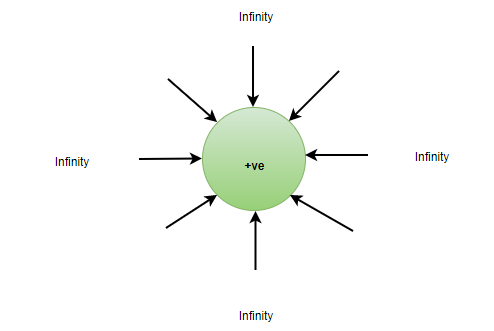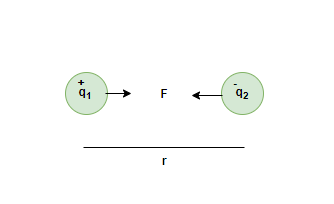# Electric Charge – Definition, History, Types and Properties

• Last Updated : 30 Jun, 2021

The most basic unit of matter was an atom, and it was proved that an atom can not be broken into smaller pieces. It was later proved that an atom can be further broken into electrons, protons, and neutrons. Electron and Proton are the negative and positive charges respectively. The presence of these charges can be seen and noticed in everyday life as well. For instance, if a scale/balloon is rubbed on dry hair, or kept near it, it starts to attract those hair strands. This is due to the fact that a balloon and dry hair are both oppositely charged.

### History of Electric Charge

The word Electricity has come from the word electron which came from a Greek word named “Elecktron” which means Amber in Greek. In 1600, William Gilbert, a philosopher mentioned “The Amber Effect” in his book which explained how the mineral Amber which is used to make jewelry keeps on getting small fibers of cloth attached to it making it difficult to wear as an ornament.

Attention reader! All those who say programming isn't for kids, just haven't met the right mentors yet. Join the  Demo Class for First Step to Coding Course, specifically designed for students of class 8 to 12.

The students will get to learn more about the world of programming in these free classes which will definitely help them in making a wise career choice in the future.

Later in the 18th century, Benjamin Franklin conducted experiments and deduced that there exist charges, and named charges as positive and negative. The concept of charges completely came into the picture after batteries were introduced.

### Electric Charge

Electric charge is that property of matter which causes the matter to experience a certain force when placed in an electric field. Static charges produce Electric Field and when these charges start to move and become dynamic, they produce a magnetic field as well, moving charge is responsible for the production of electricity as well, to be precise, it is the movement of electrons that produces electricity.

Unit of Charge

The SI unit of Electric Charge is Coulomb, and it is represented as ‘C’. The value present on a single charge (whether positive or negative) is 1.6× 10-19C.

Formula

If the charge flowing inside a circuit producing electric current is needed to be calculated, the formula used for the same will be,

q = I × T

Where, I = current flowing inside the circuit

T = Time for which the current is flowing.

Defining 1 Coulomb charge

1 Coulomb of charge can be defined as the amount of charge transferred in one second.

### Types of Electric Charge

There are only two types of electric charges present, positive charge, and negative charge. They both have the same amount of charge present on them, but with opposite signs.

Positive Charge

Positive charges are also known as Protons and the electric field lines come out of the positive charge. The charge present on a proton is +1.6 × 10-19C. If an object is positively charged, it can be concluded that the object has more protons than electrons.Electric field lines coming out of a positive charge

Negative Charge

Negative charges are also known as electrons. The electric field lines come from infinity inside a negative charge. The amount of charge present on an electron is -1.6 × 10-19C. If an object is known to be negatively charged, that means that the object has more electrons than protons.Electric field lines shown on a negative charge

Note:

One of the important properties of electric charge states that like charges (Positive and positive charge, or negative and negative charge) repel each other while unlike charge (a positive and a negative charge) attract each other.

### Properties of Electric Charge

In an Isolated system, the total charge present inside the system is the algebraic sum of all the charges present (keep in mind that the sign of the charge is to be taken into account while adding).

Q = q1+ q2+ q3+….. qn

• Conservation of Charges

It is said that the charges are always conserved. This means that the charges can neither be created nor destroyed. The charges can be transferred from one body to another, and they can flow in a closed circuit.

• Quantization of Charge

Electric charges can not be defined in decimals. They are always present as the integral multiple of them. Therefore, in any system, charges are defined as,

q = ne

Where, n = Integer numbers

e = value of the charge (1.6× 10-19 C)

### Coulomb’s Law

Coulomb’s Law is used to define the Force between two charges. Two charges can either have a force of attraction or a force of repulsion between them.

Let’s say that there are two charges placed at a distance of ‘r’. The charges are named q1 and q2, and they are stationary charges placed in vacuum/Free Space,Force will be defined as,Where,is a ConstantHere, εo = 8.854× 10-12 C2/N-m2

Force between two charges q1 and q2 when they are placed in any other medium than Free Space,Where, ε = Absolute permittivity

If the ratio of the force in the free space and force in the medium is taken out, the relationship between absolute permittivity and relative permittivity (εr) is found out,

ε/εo = εr

εr = Relative permittivity or Dielectric constant

### Sample Problem

Question 1: How many electrons are present in 1 Coulomb of charge?

Solution:

In a single electron, charge is equal to 1.6 × 10-19C

1.6× 10-19C = 1 electron

1C = 1/(1.6 × 10-19) electrons

1C = 0.625 × 1019 electrons

1C = 6.25 × 1018 electrons

Question 2: Is Electric Charge a scalar quantity or a vector quantity?

Electric charge is a Scalar quantity and this means that electric charge has magnitude but no direction. The additivity property of electric charge is a good way to prove that charges are scalar in nature. The algebraic sum do not take into account the directions of any charge placed in the system.

Question 3: How many Protons are present in 10 C of charge?

Solution:

According to the Quantization of charge,

Q = ne

Q = 10C, n = number of protons, e = 1.6 × 10-19C

10 = n × 1.6× 10-19

n = 625 × 1017 protons.

Question 4: In a system, the charges present are +5C, -7C, +1C, +9C, -15C. What is the total charge on the system?

Solution:

According to the Additivity property of Electric charges,

Q = q1+ q2+ q3+ q4+ q5

Q = +5C + (-7C) + 1C+ 9C+ (-15C)

Q = -7C

Question 5: Two charges +5C and +3C are placed at 1 meter apart in Free Space. Find the Force acting on them, state the type of force as well.

Solution:

Force acting on +5C and +3C in free space is given by,Since, the charge +5C and +3C are alike in nature, that is, they are both positive, hence, the force acting between them is the Repulsive Force.

My Personal Notes arrow_drop_up